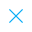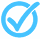Call Support +91-85588-96644

Keep me logged in
You can't leave Captcha Code empty
By submitting this form, you agree to the Terms & Privacy Policy.
ORTests given# Statistics - 1 - M (X)

Topics Covered:Calculating Mean from its DefinitionCalculation of Mean and ModeCalculating Mean by Assumed Mean MethodCalculating Mean From its DefinitionCalculating Mean by Assumed Mean MethodCalculating Mean by Step Deviation MethodCalculating Mean by Step Deviation MethodCalculating Mean of Grouped Data

This pdf is currently unavailable.